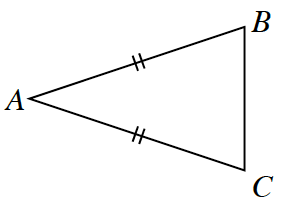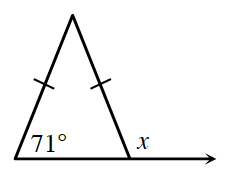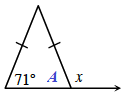### Home > CCG > Chapter 2 > Lesson 2.1.2 > Problem2-20

2-20.

In problem 2-11, you determined that because an isosceles triangle has reflection symmetry, then it must have two angles that have equal measure.

Think about what the marks on the sides of the triangles mean, and how that relates to the angles.

1.How can you tell which angles have equal measure? For example, in the diagram at right, which angles must have equal measure? Name the angles and explain how you know.

Angle $B$ is congruent to angle $C$, because the line of symmetry must pass through $A$ and these angles are on opposite sides of the line of symmetry.

2. Examine the diagram for part (a).  If you know that $m∠B+m∠C=124º$, then what is the measure of $∠B$?  Explain how you know.

1.Use this idea to find the value of $x$ in the diagram at right.  Be sure to show all work.

• Remember that $A$ and $x$ are supplementary angles whose sum is $180º$.